## Radiation in Atoms

The interaction of atoms with electromagnetic waves can be computed using time dependent perturbation theory. The atomic problem is solved in the absence of EM waves, then the vector potential terms in the Hamiltonian can be treated as a perturbation.In a gauge in which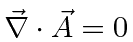, the perturbation isFor most atomic decays, theterm can be neglected since it is much smaller than the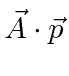term. Both the decay of excited atomic states with the emission of radiation and the excitation of atoms with the absorption of radiation can be calculated.

An arbitrary EM field can be Fourier analyzed to give a sum of components of definite frequency. Consider the vector potential for one such component,. The energy in the field is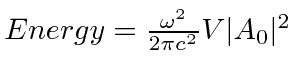. If the field is quantized (as we will later show) with photons of energy, we may write field strength in terms of the number of photons.The direction of the field is given by the unit polarization vector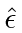. The cosine term has been split into positive and negative exponentials. In time dependent perturbation theory, the positive exponential corresponds to the absorption of a photon and excitation of the atom and the negative exponential corresponds to the emission of a photon and decay of the atom to a lower energy state.

Think of the EM field as a harmonic oscillator at each frequency, the negative exponential corresponds to a raising operator for the field and the positive exponential to a lowering operator. In analogy to the quantum 1D harmonic oscillator we replace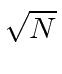byin the raising operator case.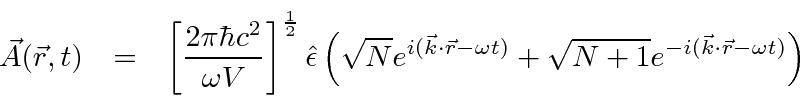With this change, which will later be justified with the quantization of the field, there is a perturbation even with no applied field ()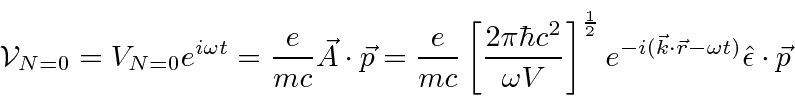which can cause decays of atomic states.

Plugging thisfield into the first order time dependent perturbation equations, the decay rate for an atomic state can be computed.The absolute square of the time integral from perturbation theory yields the delta function of energy conservation.

To get the total decay rate, we must sum over the allowed final states. We can assume that the atom remains at rest as a very good approximation, but, the final photon states must be carefully considered. Applying periodic boundary conditions in a cubic volume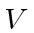, the integral over final states can be done as indicated below.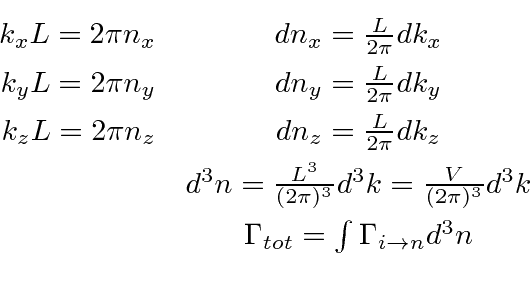With this phase space integral done aided by the delta function, the general formula for the decay rate is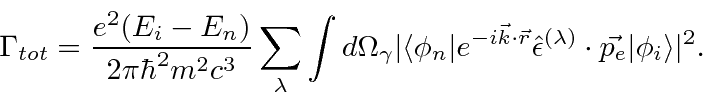This decay rate still contains the integral over photon directions and a sum over final state polarization.

Computation of the atomic matrix element is usually done in the Electric Dipole approximationwhich is valid if the wavelength of the photon is much larger than the size of the atom. With the help of some commutation relations, the decay rate formula becomesThe atomic matrix element of the vector operator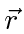is zero unless certain constraints on the angular momentum of initial and final states are satisfied. The selection rules for electric dipole (E1) transitions are:This is the outcome of the Wigner-Eckart theorem which states that the matrix element of a vector operator, where the integerruns from -1 to +1, is given by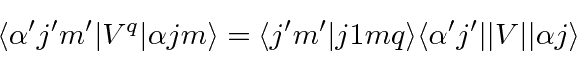Hererepresents all the (other) quantum numbers of the state, not the angular momentum quantum numbers. In the case of a simple spatial operator like, only the orbital angular momentum is involved.We derive a simple result for the total decay rate of a state, summed over final photon polarization and integrated over photon direction.This can be used to easily compute decay rates for Hydrogen, for example the 2p decay rate.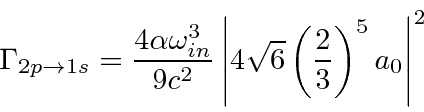The total decay rate is related to the energy width of an excited state, as might be expected from the uncertainty principle. The Full Width at Half Maximum (FWHM) of the energy distribution of a state is. The distribution in frequency follows a Breit-Wigner distribution.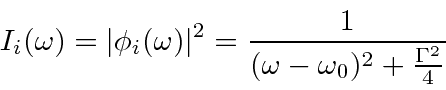In addition to the inherent energy width of a state, other effects can influence measured widths, including collision broadening, Doppler broadening, and atomic recoil.

The quantum theory of EM radiation can be used to understand many phenomena, including photon angular distributions, photon polarization, LASERs, the Mössbauer effect, the photoelectric effect, the scattering of light, and x-ray absorption.

Jim Branson 2013-04-22